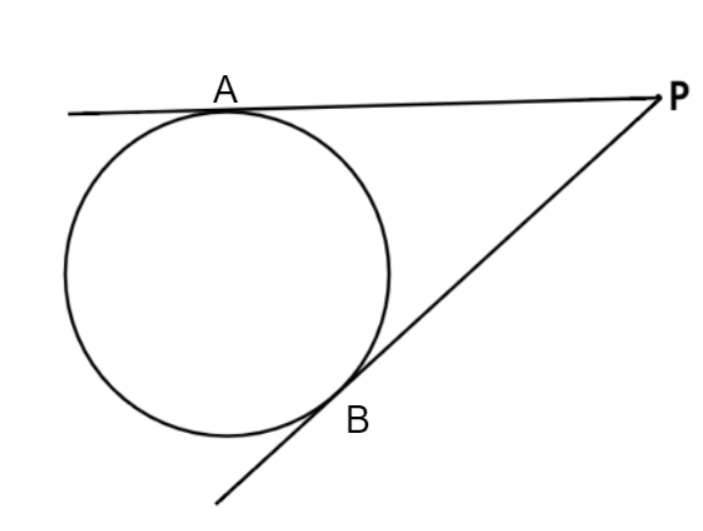Questions & AnswersQuestionAnswers

# Draw a circle with the help of a bangle. Take a point P outside the circle. Construct the pair of tangents from this point P to the circle.Answer Verified
Hint: Here, we will be using the basic geometry and will be drawing the pair of tangents from point P with the help of a scale.

Complete step-by-step answer:Step1- Firstly, we will take the bangle and draw the circle with the help of the bangle by marking the boundary of the bangle.
Step2- Now, we will take any point P outside the circle whose coordinates will be given for any particular case and then we will draw a pair of lines from this point P to the points where these lines will just touch the circle. Through this we will get a pair of tangents from point P to the circle drawn by the bangle.
Step3- Finally we can conclude that the tangents are touching the drawn circle at two points say A and B. So PA and PB are the two required pairs of tangents from point P to the drawn circle.

Note: In these type of problems, we simply take the bangle and draw the outer boundary of the bangle with the help of pencil and then mark the given point P and through that point two lines are extended such that these lines touch the circle because tangents to the circle are the lines which touches the circle.
Bookmark added to your notes.
View Notes
How to Draw a Perfect CircleTangent of a CircleTangent to a CircleConstruction of Tangent to a CircleRadius of a CircleCircumference of a CircleEquation of A CircleSecant of a CircleSector of a CircleArea of a Circle